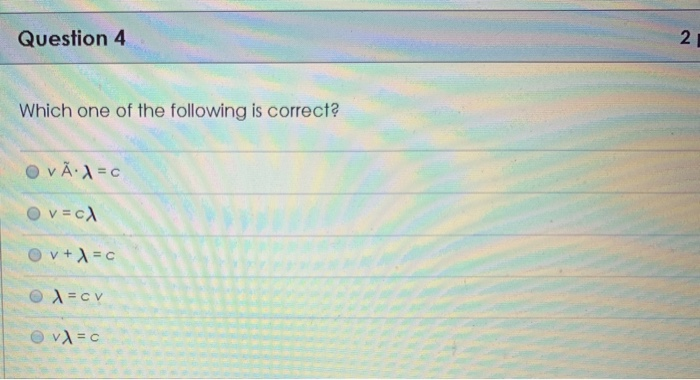# Question:2 Question 4 Which One Of The Following Is Correct? Vc Ov+c Vc

2 Question 4 Which One Of The Following Is Correct? Vc Ov+c VcSmart Teacher

Above formula is to find the relationship between frequency, wavelength and speed of light of electromagnetic waves

C= speed of light

= frequency of wave

= wavelength of the wave

according to law

frequency is inversely proportional to the wavelength

1/

= C/

= C

So the last option is correct